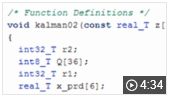# Get Started with MATLAB Coder

Generate C and C++ code from MATLAB code

MATLAB® Coder™ generates C and C++ code from MATLAB code for a variety of hardware platforms, from desktop systems to embedded hardware. It supports most of the MATLAB language and a wide range of toolboxes. You can integrate the generated code into your projects as source code, static libraries, or dynamic libraries. The generated code is readable and portable. You can combine it with key parts of your existing C and C++ code and libraries. You can also package the generated code as a MEX-function for use in MATLAB.

When used with Embedded Coder®, MATLAB Coder provides code customizations, target-specific optimizations, code traceability, and software-in-the-loop (SIL) and processor-in-the-loop (PIL) verification.

To deploy MATLAB programs as standalone applications, use MATLAB Compiler™. To generate software components for integration with other programming languages, use MATLAB Compiler SDK™.

Installation and Configuration

## VideosGenerate C Code from MATLAB Code
Workflow for generating C/C++ code from MATLAB code.

##### SupportGet trial now